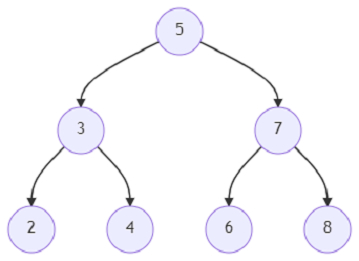# Program to change the root of a binary tree using Python

Suppose, we are given a binary tree and a node that is situated at the leaf of the binary tree.We have to make the leaf node the root node of the binary tree. We can do it in the following way −

• If a node has a left child, it becomes the right child.

• A node's parent becomes its left child. In this process, the parent node's link to that node becomes null, so it will have only one child.

The node structure of the tree is like below −

TreeNode:
data: <integer>
left: <pointer of TreeNode>
right: <pointer of TreeNode>
parent: <pointer of TreeNode>

We have to return the root of the converted tree.

So, if the input is likeand the new root is 8; then the inorder representation of the converted tree will be − 2, 3, 4, 5, 7, 6, 8,

The new root node of the tree is 8.

To solve this, we will follow these steps −

• Define a function helper() . This will take node, new_par

• if node is same as root, then

• parent of node := new_par

• if left of node is same as new_par, then

• left of node := null

• if right of node is same as new_par, then

• right of node := null

• return root

• if left of node is not null, then

• right of node := left of node

• if left of parent of node is same as node, then

• left of parent of node := null

• left of node := helper(parent of node, node)

• parent of node := new_par

• return node

• return helper(leaf, null)

Let us see the following implementation to get better understanding −

## Example

import collections
class TreeNode:
def __init__(self, data, left = None, right = None, parent = None):
self.data = data
self.left = left
self.right = right
self.parent = parent
def insert(temp,data):
que = []
que.append(temp)
while (len(que)):
temp = que
que.pop(0)
if (not temp.left):
if data is not None:
temp.left = TreeNode(data, parent = temp)
else:
temp.left = TreeNode(0, parent = temp)
break
else:
que.append(temp.left)
if (not temp.right):
if data is not None:
temp.right = TreeNode(data, parent = temp)
else:
temp.right = TreeNode(0, parent = temp)
break
else:
que.append(temp.right)
def make_tree(elements):
Tree = TreeNode(elements)
for element in elements[1:]:
insert(Tree, element)
return Tree
def search_node(root, element):
if (root == None):
return None
if (root.data == element):
return root
res1 = search_node(root.left, element)
if res1:
return res1
res2 = search_node(root.right, element)
return res2
def print_tree(root):
if root is not None:
print_tree(root.left)
print(root.data, end = ', ')
print_tree(root.right)
def solve(root, leaf):
def helper(node, new_par):
if node == root:
node.parent = new_par
if node.left == new_par:
node.left = None
if node.right == new_par:
node.right = None
return root
if node.left:
node.right = node.left
if node.parent.left == node:
node.parent.left = None
node.left = helper(node.parent, node)
node.parent = new_par
return node
return helper(leaf, None)
root = make_tree([5, 3, 7, 2, 4, 6, 8])
root = solve(root, search_node(root, 8))
print_tree(root)

## Input

root = make_tree([5, 3, 7, 2, 4, 6, 8])
root = solve(root, search_node(root, 8))

## Output

2, 3, 4, 5, 7, 6, 8,